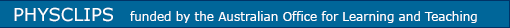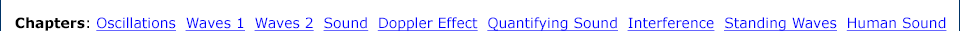Links to related material and teachers' resources Travelling waves, superposition, reflection and transmission Wave pulses in a stretched string. Equations for a travelling wave. Linear media. Superposition. The limits of linearity. Reflections at fixed and free boundaries. Reflection and transmission at step changes in density.The travelling sine wave Describing the travelling sine wave. Comoving and fixed coordinates. y = sin (kx − ωt). Three dimensional plots. Phases in a travelling wave.Moving frames of reference Vector addition and subtraction. Position, velocity and acceleration in different frames.Oscillations Inertia plus a restoring force produces oscillations. The mechanical equation for Simple Harmonic Motion. Initial conditions. Cyclic and angular frequency. Mechanical and kinetic energies. The simple pendulum. A nonlinear pendulum. Damped oscillations. Forced oscillations. Resonance in one, two and three dimensions.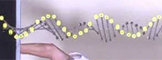Simple Harmonic Motion Simple Harmonic Motion as a projection of circular motion. Displacement, velocity and acceleration. Chladni patterns and sinusoidal vibration.The Mexican Wave The mexican wave (or stadium wave) is an example of a travelling wave.Laboratory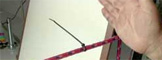Downloads (thumbnails at 50% of size of animation)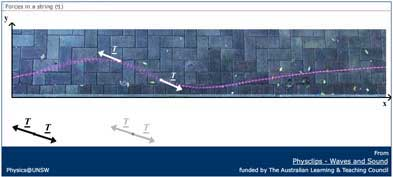Download (.zip) Download (.zip)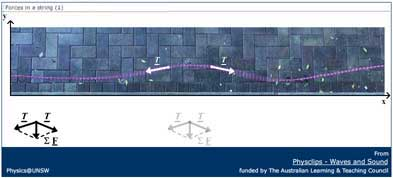Download (.zip) Download (.zip)Download (.zip) Download (.zip)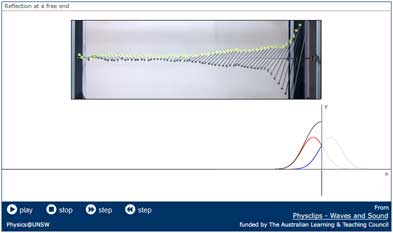Download (.zip) Download (.zip)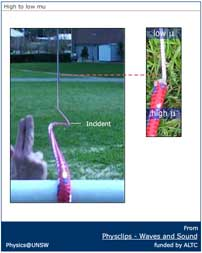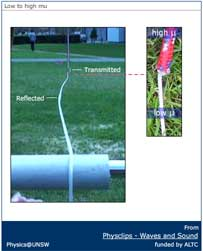Download (.zip) Download (.zip)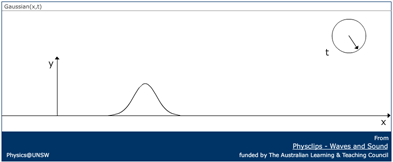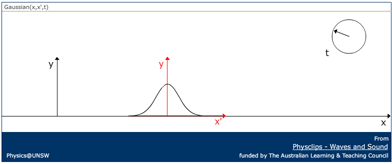Download (.zip) Download (.zip)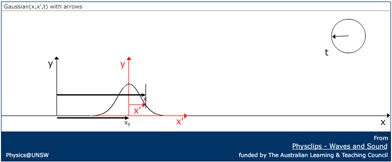Download (.zip) Download (.zip)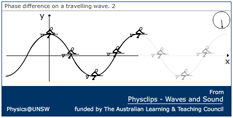Download (.zip) Download (.zip)